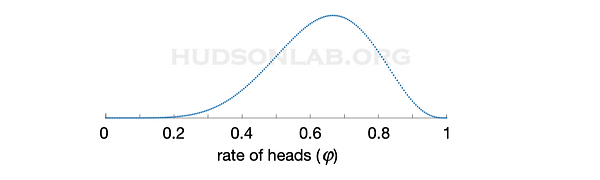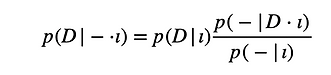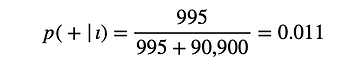top of page

#### What is probability?

• How are probabilities written?

• What is probability?

• What is Bayes’ Theorem?

• How are probabilities computed?

• How are probabilities used to test hypotheses?

• What are the drawbacks of the Bayesian definition of probability?

Here we will answer the question ‘what is probability’ from the original (as conceptualized by the originators of probability theory, such as Thomas Bayes, James Bernoulli, Blaise Pascal and Pierre Simon Laplace) Bayesian perspective.

How are probabilities written?

It is standard to talk about probabilities in terms of flipping a coin, so let’s go ahead and start there. To express a coin probability, we have to first define certain propositions (statements) about the coin and our background information about the scenario. For example, our background information might include something like the propositions:We also need to know what probability we want to compute, which we’ll call the object of the probability statement. An example might be:Probability statements are written in the particular format given in the figure, so that we can immediately see the separation between the object and background information, separated by the vertical line. The statement in the figure is read, ‘the probability of x, given either y or z, and iota. The propositions in the background information (to the right of the vertical line) are also called conditioning statements, because they define the conditions we assume are true when we compute the probability of the object. In the case of coin-flipping, we might want to compute the probability:In all cases, we are computing a numerical value for the object of the probability statement (propositions to the left of the vertical bar), in the scenario defined by the conditioning statements. The numerical values of the probabilities we compute are only valid when the conditioning statements are true.

What is probability?

So far, we’ve seen how to write probabilities, but we still haven’t seen a definition of probability.

Here we will answer the question ‘what is probability’ from a Bayesian perspective. Within the Bayesian tradition probabilities were defined in the 17th century as ‘a degree of belief’.

• Although this definition may sound antiquated to the modern ear, it probably would have have been expressed today as ‘the degree to which your information suggests that a proposition (such as ‘it will rain tomorrow’) is true’.

• Expressed as a measure of the available information (that compels us to assert the truth or falsity of a proposition) should make it clear that this definition of probability is entirely modern and scientific ​​
• in particular, it is objective in the scientific sense of agreement across individuals

• if two individuals have the same information, they must assert the same probability assignments with regard to their shared information ​

This definition of probability covers any proposition, whether or not it can be expressed as a frequency. Thus, if we were somehow in possession of the infinite series of coin-flips necessary to define probabilities within the frequentist tradition, we could use that information to assign coin probabilities. However, if we were in possession of doppler radar, barometric pressure, humidity and information from historical precipitation trends, we could use that information to assign a probability that it will rain tomorrow - despite this being a singular event that could not recur.

Most importantly, we can use this definition to assign probabilities to scientific hypotheses. The probability that ‘a given hypothesis is correct’ is based on our information regarding the truth or falsity of that hypothesis. It can therefore change based on new information. Further, when we base the probabilities of hypotheses on experimental data we bring our information, and therefore our probability assignments, into register with the world.What is Bayes’ Theorem

Bayes’ theorem is the foundation of modern probability theory, in the sense that we start most probability computations from this equation. In a scientific context, Bayes’ theorem can be written:

How are probabilities computed?

​In general, there are two routes to assigning probabilities, positive knowledge and experimental data, or using arguments from ignorance.

Arguments from ignorance usually serve as constraints on the probability assignments that could be made in an internally consistent way.

For example, if you are about to flip a coin but have not yet seen any previous flips (data), what would your best guess for the rate of heads be? Before you have seen any data, your information about potential heads and tails outcomes is symmetrical: without more information, any argument you can make for predicting heads should also apply to predicting tails.

Based on this symmetry in your ignorance about heads and tails outcomes, you have to assign the two outcomes equal probability. The only guess about the probability of observing heads on the next coin-toss that can be equal to the probability of observing tails on the next coin-toss is to assign a probability of 0.5 to each.where h is the number of heads outcomes and n is the total number of coin-flips. For the dataset  d = [h,h,h,t,h,t,h,h,t], this yields the distribution shown in Fig. 2.

>> thetas=linspace(0,1,201); p=nchoosek(9,6)*(thetas.^6).*(1-thetas).^3;

>> figure; subplot(2,1,1); plot(thetas,p,’.')Probabilities are manipulated via the two rules of probability theory:

(1) The sum rule:which becomes:when the propositions x and y are non-overlapping.

(2) The product rule:Bayes’ theorem is a straightforward consequence of the product rule, because:How are probabilities used to test hypotheses?

One of the more memorable ways in which people encounter probabilities is after having a diagnostic test in a hospital.

• Here, the outcome of the diagnostic test is the experimental datum that will help us decide whether a disease is present (D) or absent (~D).
• The outcome of the diagnostic test can either be positive (+) or negative (-)

• The combinations of D/~D and +/- cases are shown in the contingency table:The probability of having the disease on getting a positive diagnostic test is written:whereas the probability of having the disease after receiving a negative diagnostic test is:Notice that in both cases, the iota term encodes your background information regarding the disease D and the properties of the diagnostic test.

According to the table, this disease has a low prevalence in the general population

i.e., it has a low prior probability, since:Further, the we can compute the likelihood terms,called the sensitivity and specificity of the test in medical circles.

• The sensitivity of the test tells you how often the test correctly identifies the disease (95 times) when it is in fact present (out of 100 disease-present cases)

• The specificity tells you how often the test correctly rules the disease out (90,000 times) when it is in fact not present (out of 90,900 total disease-absent cases)

The final calculation also requires that we know the overall rate at which the test gave:

• positive test results:• negative test results:Thus, the total calculations are :

1These numbers tell an interesting story. If you get a negative test result, it is extremely unlikely that you have the underlying disease, which is indicative of a ‘good’ test (high sensitivity and specificity). However, if you get a positive test result, you still have a very low probability of actually having the underlying disease (only about 10%).

The reason is that the disease is just very uncommon (low prevalence), and so it’s unlikely for anyone to have it, regardless of the test result. The test is still quite good, and of course you are over 1000x more likely to have the disease if you’ve had a positive test result vs. a negative one.

What are the drawbacks of the Bayesian definition of probability?

The major drawback of the Bayesian approach is practical, rather than theoretical: it is far more mathematically intensive than the frequentist approach. Computing posterior probabilities for a set of hypotheses (such as competing scientific hypotheses),usually requires a more complex version of Bayes’ theorem:where d is the observed data,• Gaussian variance is a common exampleIn this more complex case, we must integrate over any unknown parameters (in this example, phi) with respect to the prior probability over its possible parameter values. These integrals will often put Bayesian data analysis outside the realm of the undergraduate classroom.

Indeed, it is often the case that no analytical solution exists for the integrals needed for computing Bayesian posteriors, and approximate computational techniques must be devised in their stead.

bottom of page